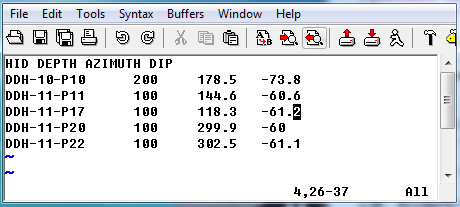# 5.1.11. Borehole survey file format¶

GIFtools assumes that the borehole survey file is in either the XYZ or CSV file format. If the file is not given during the borehole data import, than GIFtools assumes the boreholes are vertical. The file must have the at least the following information (but not necessarily these specific names or order):

1. Hole_ID: If the hole ID names require a space, then a CSV file should be used. These identifications can be numeric or characters (with no quotes), but should be consistent with the collar and property files.

2. Length: Length of observation down the borehole

3. Azimuth: Azimuth of the borehole trace at the downhole length in degrees. 90\(^o\) is North.

4. Dip: Dip of the borehole trace at the downhole length in degrees. Dip is from horizontal: -90\(^o\) is vertical and 0\(^o\) is a horizontal trace.

## 5.1.11.1. Example¶

Below is a sample survey file (XYZ format) where “HID” is the Hole_ID and “DEPTH” is the Length: